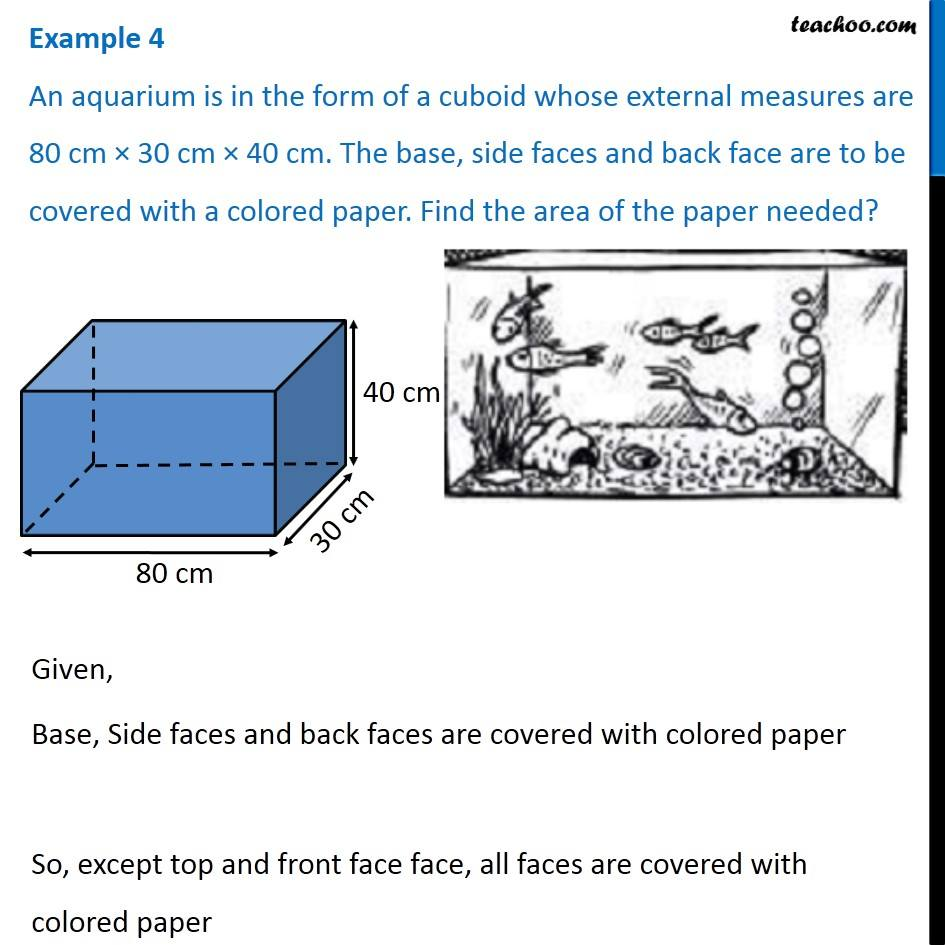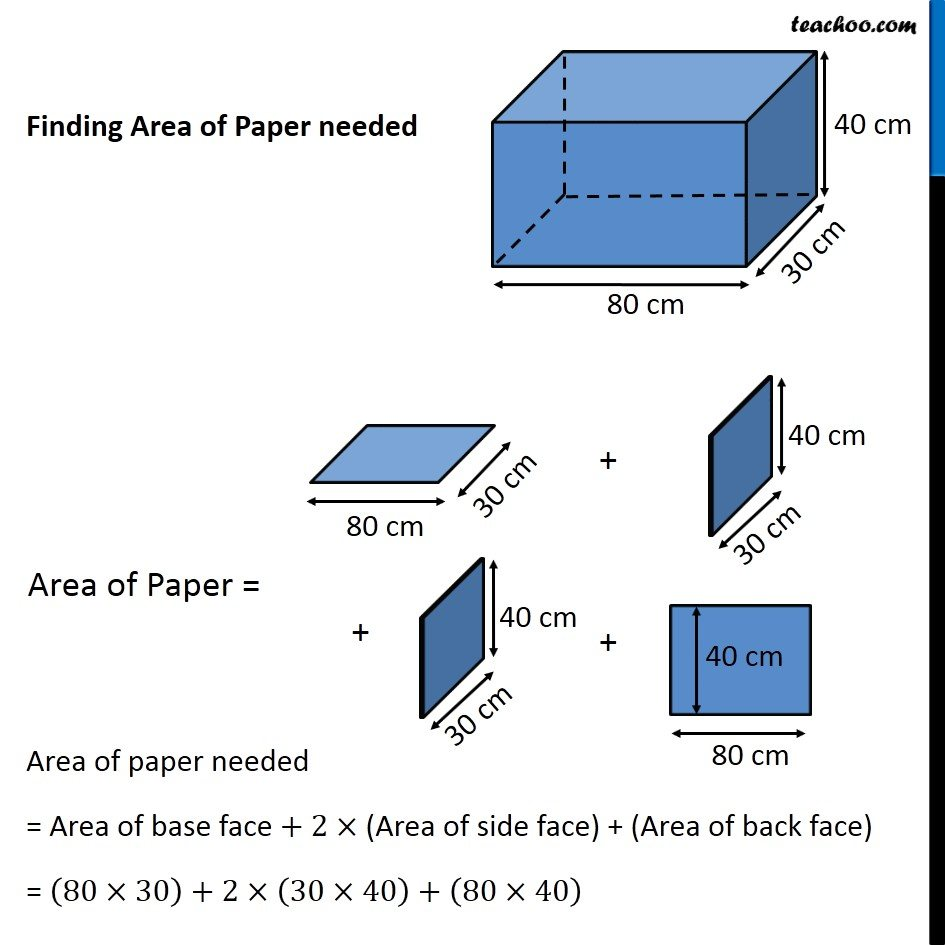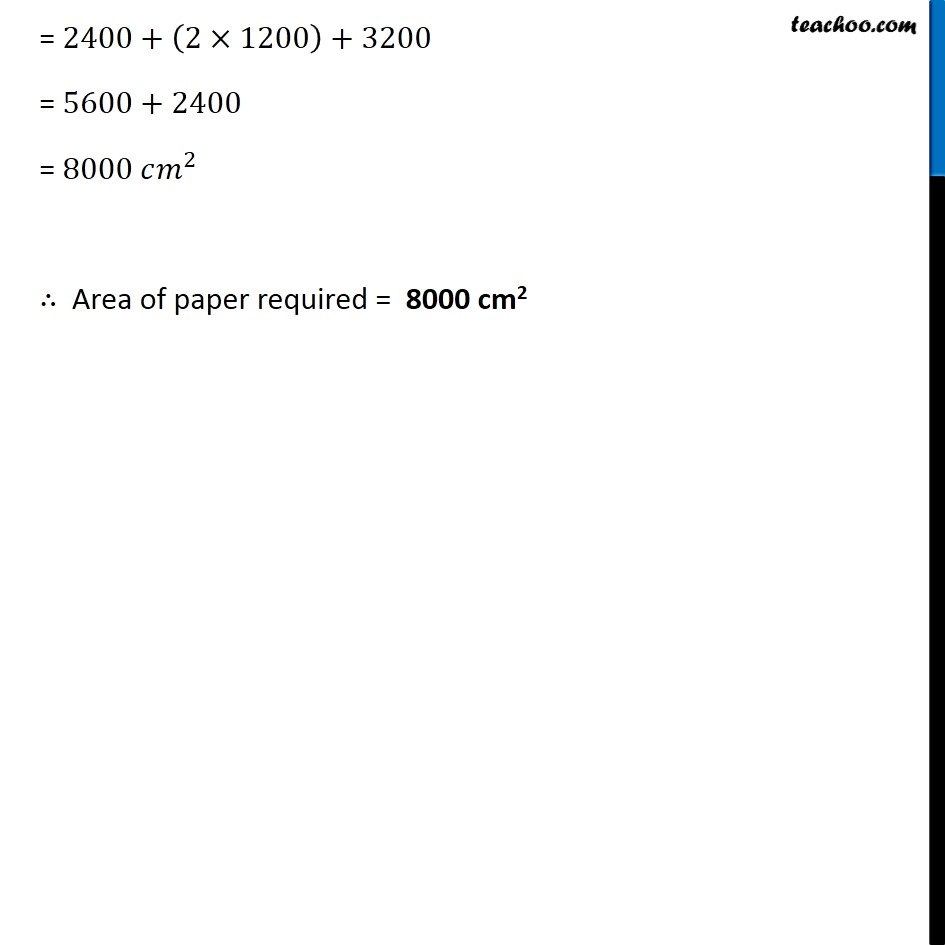1. Chapter 11 Class 8 Mensuration
2. Serial order wise
3. Examples

Transcript

Example 4 An aquarium is in the form of a cuboid whose external measures are 80 cm × 30 cm × 40 cm. The base, side faces and back face are to be covered with a colored paper. Find the area of the paper needed? Given, Base, Side faces and back faces are covered with colored paper So, except top and front face face, all faces are covered with colored paper Finding Area of Paper needed Area of Paper = Area of paper needed = Area of base face + 2× (Area of side face) + (Area of back face) = (80×30)+2×(30×40)+(80×40) = 2400+(2×1200)+3200 = 5600+2400 = 8000 𝑐𝑚^2 ∴ Area of paper required = 8000 cm2

Examples

Chapter 11 Class 8 Mensuration
Serial order wise